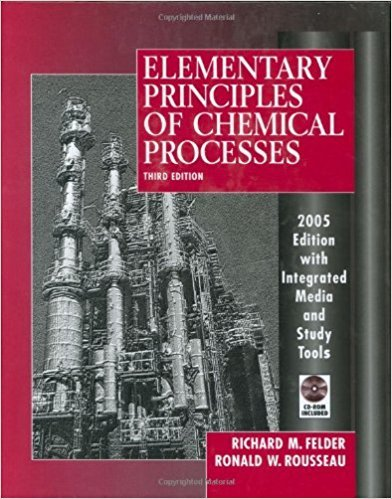×
Get Full Access to Elementary Principles Of Chemical Processes - 3 Edition - Chapter 9 - Problem 9.12
Get Full Access to Elementary Principles Of Chemical Processes - 3 Edition - Chapter 9 - Problem 9.12

×

# In the production of many microelectronic devices,ISBN: 9780471687573 143

## Solution for problem 9.12 Chapter 9

Elementary Principles of Chemical Processes | 3rd Edition

• Textbook Solutions
• 2901 Step-by-step solutions solved by professors and subject experts
• Get 24/7 help from StudySoup virtual teaching assistantsElementary Principles of Chemical Processes | 3rd Edition

4 5 1 387 Reviews
12
4
Problem 9.12

In the production of many microelectronic devices, continuous chemical vapor deposition (CVD) processes are used to deposit thin and exceptionally uniform silicon dioxide films on silicon wafers. One CVD process involves the reaction between silane and oxygen at a very low pressure. S~(g) + Oz(g) -- SiOz(s) + 2 Hz(g) The feed gas, which contains oxygen and silane in a ratio 8.00 mol 02/mol SiH4, enters the reactor at 298 K and 3.00 torr absolute. The reaction products emerge at 1375 K and 3.00 torr absolute. Essentially all of the silane in the feed is consumed. (a) Taking a basis of 1 m3 of feed gas, calculate the moles of each component of the feed and product mixtures and the extent of reaction, Hmol). (b) Calculate the standard heat of the silane oxidation reaction (kllmol). Then, taking the feed and product species at 298 K (25C) as references, prepare an inlet-outlet enthalpy table and calculate and fill in the component amounts (mol) and specific enthalpies (kllmol). (See Example 9.5-1.) Data (IlH~)siH4(g) = -61.9 kllmol, (IlH~)si02(S) = -851 kllmol (CphiH4(g)[kJ/(mol'K)] = 0.01118 + 12.2 X 1O- 5 T - 5.548 X 1O- 8 T2 + 6.84 X 1O- 1Z T3 (Cp )sio2(s)[kJ/(mol'K)] = 0.04548 + 3.646 X 10-5T -1.009 X 103 /T2 The temperatures in the formulas for Cp are in kelvin. (c) Calculate the heat (kJ) that must be transferred to or from the reactor (state which it is). Then determine the required heat transfer rate (kW) required for a reactor feed of 27.5 mJIh.

Step-by-Step Solution:
Step 1 of 3

Step 2 of 3

Step 3 of 3

##### ISBN: 9780471687573

Elementary Principles of Chemical Processes was written by and is associated to the ISBN: 9780471687573. The full step-by-step solution to problem: 9.12 from chapter: 9 was answered by , our top Chemistry solution expert on 11/15/17, 02:42PM. This full solution covers the following key subjects: feed, mol, reaction, calculate, kllmol. This expansive textbook survival guide covers 13 chapters, and 710 solutions. This textbook survival guide was created for the textbook: Elementary Principles of Chemical Processes, edition: 3. Since the solution to 9.12 from 9 chapter was answered, more than 421 students have viewed the full step-by-step answer. The answer to “In the production of many microelectronic devices, continuous chemical vapor deposition (CVD) processes are used to deposit thin and exceptionally uniform silicon dioxide films on silicon wafers. One CVD process involves the reaction between silane and oxygen at a very low pressure. S~(g) + Oz(g) -- SiOz(s) + 2 Hz(g) The feed gas, which contains oxygen and silane in a ratio 8.00 mol 02/mol SiH4, enters the reactor at 298 K and 3.00 torr absolute. The reaction products emerge at 1375 K and 3.00 torr absolute. Essentially all of the silane in the feed is consumed. (a) Taking a basis of 1 m3 of feed gas, calculate the moles of each component of the feed and product mixtures and the extent of reaction, Hmol). (b) Calculate the standard heat of the silane oxidation reaction (kllmol). Then, taking the feed and product species at 298 K (25C) as references, prepare an inlet-outlet enthalpy table and calculate and fill in the component amounts (mol) and specific enthalpies (kllmol). (See Example 9.5-1.) Data (IlH~)siH4(g) = -61.9 kllmol, (IlH~)si02(S) = -851 kllmol (CphiH4(g)[kJ/(mol'K)] = 0.01118 + 12.2 X 1O- 5 T - 5.548 X 1O- 8 T2 + 6.84 X 1O- 1Z T3 (Cp )sio2(s)[kJ/(mol'K)] = 0.04548 + 3.646 X 10-5T -1.009 X 103 /T2 The temperatures in the formulas for Cp are in kelvin. (c) Calculate the heat (kJ) that must be transferred to or from the reactor (state which it is). Then determine the required heat transfer rate (kW) required for a reactor feed of 27.5 mJIh.” is broken down into a number of easy to follow steps, and 255 words.

Unlock Textbook Solution